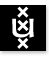Advanced Topics in Recursion Theory 2004/2005; 2nd Semester Institute for Logic, Language & Computation Universiteit van Amsterdam
Instructor: Dr Benedikt Löwe
Vakcode: MolATRT6
Time: Thursday, 17-19
Place: P.018
Grader: Dipl.-Math. Stefan Bold (sbold (at) science.uva.nl)
Course language: English
Intended Audience: M.Sc. students of Logic and Mathematics
Prerequisites: "Recursion Theory" (as taught in the first semester); the basic theory of Turing degrees and recursively enumerable sets.

This course will start where Recursion Theory ended in the first semester: with the Kleene-Post theorem (Theorem 10.6.3 in Cooper's book). Starting from there, we shall discuss more advanced recursion-theoretic constructions (finite injury arguments and infinite injury arguments) and then discuss some ideas of infinitary computation.

Literature: Barry Cooper, "Computability Theory" (Chapters 10, 12, 13 and 14). We will sell the book to enrolled students for EUR 32 in the break of the first lecture (at a substantial discount compared to the list price of \$69.95). Several papers on Infinite Time Turing Machines will be handed out in class.

### Classes:

• First lecture (February 10th, 2005). Nonlinearity of the Turing degrees and nonlinearity of the c.e. degrees (Theorem 10.6.3 and Corollary 10.6.4). Definition of low and high degrees (Section 12.1).
• Second lecture (February 17th, 2005). Information content of approximations. The Friedberg Jump Inversion Theorem (Theorem 10.6.9). Principal functions. Immunity, hyperimmunity and hyperhyperimmunity (Section 12.2).
Homework (due on February 24th): Exercise 10.6.7 and Exercise 12.2.10.
• Third lecture (February 24th, 2005). Cohesiveness and its relation to immunity properties. Computation functions. The Domination Lemma. Properties definable in the language of partial orders. Definability of "hyperimmune".
Homework (due on March 10th): Exercise 12.2.16, 12.2.21, 12.2.24.
• Class cancelled (March 3rd, 2005).
• Fourth lecture (March 10th, 2005). Maximality (Theorem 12.2.27). Martin's Theorem (Every maximal set is high). Existence of a cohesive set.
• Fifth lecture (March 17th, 2005). Existence of uncountably many automorphisms of the lattice of c.e. sets. Priority method: Friedberg-Muchnik theorem.
Homework (due on April 7th): Exercise 12.2.17, 12.2.18, 12.2.32, 12.3.5.
• Sixth lecture (March 24th, 2005). The Permitting Lemma (without proof). Relative Friedberg-Muchnik without oracle (Corollary 12.3.4). Differences of c.e. sets.
• Seventh lecture (April 7th, 2005). Harrington's tree of strategies. Differences of c.e. sets and approximations. Existence of a d-c.e. degree which is not c.e.
Homework (due on April 14th): Exercise 12.3.7.
• Eighth lecture (April 14th, 2005). Sack's splitting theorem. Sacks restraints. Lattice embeddings into the c.e. degrees. The minimal pair theorem. Preparations for the proof.
• Ninth lecture (April 21st, 2005). Proof of the minimal pair theorem.
• Tenth lecture (April 28th, 2005). Forcing. Generic sets. Genericity Theorem. Nondefinability of generic sets. Every generic set is immune. 1-genericity.
Homework (due on May 12th): Exercises 13.1.4, 13.1.8, 13.2.23.
• Ascension Day (May 5th, 2005).
• Eleventh Lecture (May 12th, 2005).
Homework (due on May 19th): Exercises 13.3.5, 13.3.13.
• Twelfth Lecture (May 19th, 2005).
Homework (due on May 26th, either by e-mail to sbold@science.uva.nl or in the mailbox S.Bold): Exercise 14.3.2.

Last update : May 19th, 2005